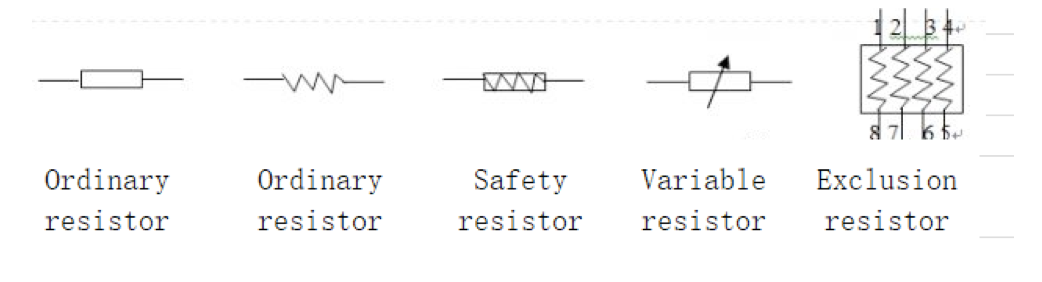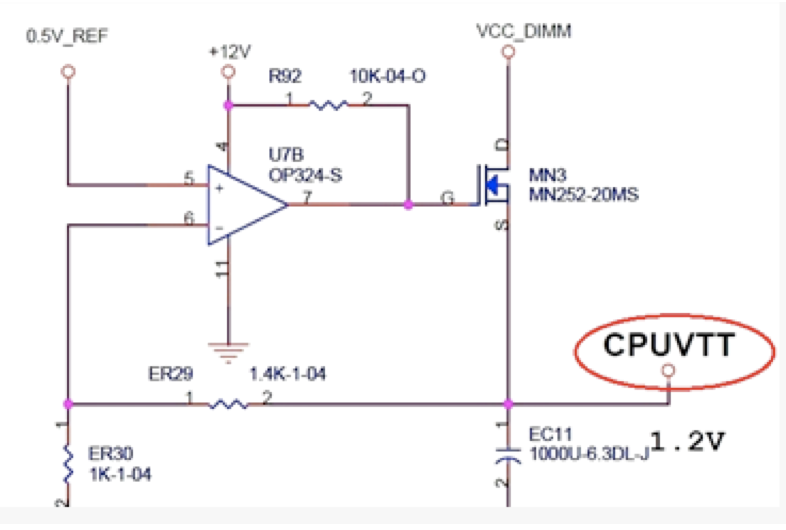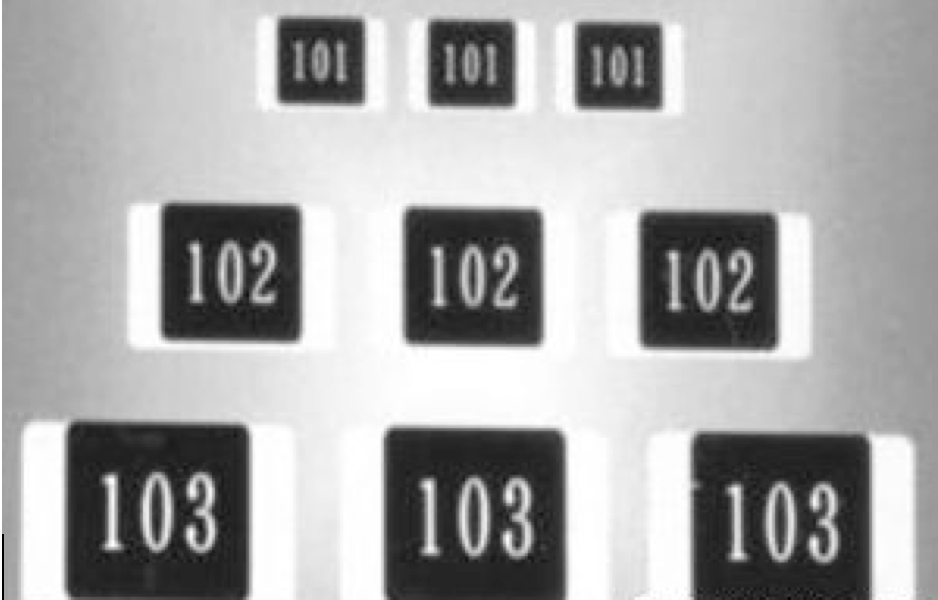### The Guide to SMT Material Packaging: Chip Resistor

Jan 7, 2019 8:00:00 PM

The obstruction effect of an object on the passage of electric current is called resistance. Anything that made specifically to be such an obstruction is simply called a resistor. Resistors are the most widely used components in circuit components and their quality has a great impact on the stability of circuit operation. Resistors are mainly used to stabilize and regulate the current and voltage in the circuit, that is, to reduce/divide voltage, to limit/shunt/isolate/filter current (in combination with a capacitor), and in matching/adjusting signal amplitude.Resistors are the most basic and most commonly used electronic components of electronic circuits. Resistors are generally represented by letter symbols such as R, RN, RF, FS, PR in electrical schematic drawings. The resistance value marked on the resistor is the nominal resistance. The basic unit of resistance is ohms, expressed in Ω. In practice, thousands of ohms (KΩ) and megohms (MΩ) are also commonly used. For reference, conversion relationship between these are similar to the metric system and not like computer memory. The conversions are  1 KΩ = 1000 Ω  and 1 MΩ = 1000 KΩ = 1000000 Ω (as examples).## 1. Insurance resistor

Fusible resistors can also be called a fuse resistor. The fuse resistor has a dual function. Under normal conditions, it has the electrical characteristics of a common resistor. Once the voltage in the circuit rises, the current increases or a component is damaged, the fuse resistor is blown for a specified period of time. This protects the circuit from damage. There are mainly chip-type fuse resistors in the computer motherboard, which are mainly used for power supply of the interface circuit. The fuses in the circuit generally are chip type, black exclusion, gray in-line and PTC. In the circuit, the fuse resistor is indicated by F. Of course, there are many 0Ω resistors in the circuit, which also acts as insurance for keeping things regulated.

## 2. Row resistor

A row resistor is a combined resistor that is integrated with discrete resistors arranged in a regular pattern. This is also known as an integrated resistor. It is a resistor network with small size, regularization, and high precision. It is suitable for electronic equipment and computer circuits. In the circuit, the row resistance is generally expressed in RN. The main resistance of the main board in the main board is 8 pin resistance. Generally, the pull-up resistor or the lower resistor is used on the data transmission line.

## 3. Direct Resistor Markings

The straight-label method refers to directly marking the type of the resistor, the nominal resistance value, the allowable error, the rated power, and other parameters on the surface of the resistor. Such as the resistance marked: 47KΩ ± 5% or 3.3Ω. It can also be represented by a number plus a character: 4K7 means 4.7KΩ or 4Ω7 means 4.7Ω## 4. Numerical Resistor Markings

The numerical method mainly has three digits indicating the resistance value, the first two digits represent the significant digits, and the third digit indicates the multiplier (10 squares). For example, the label is 472, indicating that the resistance value is: 47×102=4700Ω, labeled 330. Indicates that the resistance value is: 33 × 100 = 33 Ω. If some fuse resistors are marked as 000, they measure 0 Ω.